维纳滤波法模糊图像处理_matlab维纳滤波处理图像运动模糊 - CSDN
• 曾经很长一段时间， 对图像模糊都有一种偏见， 认为这是一个灌水的领域， 没有什么实用价值，要到这样的文章，不管是多高的档次， 直接pass。 最近在调研最近几年的关于Computational Photography的一些研究热点时...

原文：原文地址

曾经很长一段时间， 对图像去模糊都有一种偏见， 认为这是一个灌水的领域， 没有什么实用价值，要到这样的文章，不管是多高的档次， 直接pass。 最近在调研最近几年的关于Computational Photography的一些研究热点时， 发现图像去模糊这个领域非常活跃， 一些效果图还是蛮有意思的。 于是大概浏览了其中的几篇文章， 慢慢地我的这种偏见也消失了。 当数码消费电子日益普及的时候， 这项技术确实非常有用。它能挽救相当一批由于曝光时间过长或者拍摄
时手的抖动造成的模糊照片。

图像的模糊按照模糊核的性质来分类可分为： Blind image deconvolution（BID
芒去卷积）和Non-blind image deconvolution（NBID 非芒去卷积）。BID就是在模糊核未知的情况下恢复出清晰的图像。在这种情况下， 除了采集到的图像， 其他的没有任何任何信息。 NBID是在模糊核已知的情况下恢复出清晰的原始图像。 因为有了模糊核这个非常重要的信息， 去卷积的工作就相对来说容易多了， 主要任务就是如何在保持细节的情况下抑制噪声。过去三四十年， 很多研究都集中在这个领域，
也有了不少很实用的方法，比如维纳滤波， Richardson-Lucy方法。后来有些方法是基于去噪方法的， 比如傅立叶，小波，Curvelet，和TV方法。
一般来说， NBID是BID的基础。 一旦模糊核估计出来， 所有的NBID方法都可以在BID中使用。

按照模糊的区域来划分， 又可分为：全局模糊和局部模糊。 全局的模糊主要是由于拍摄设备的运动造成的，
而局部模糊是由于单个物体的 运动造成的。 从实用性来说， 全局模糊要好于局部模糊。 理由有三： （1）全局模糊可以用一个模糊核来恢复， 因此最主要的任务就是PSF的估计， 而局部模糊的模糊核是随位置变化的， 难道要大很多，结果也就不稳定很多。（2）造成全局模糊的原因是手持拍摄设备在曝光时间比较长的情况下进行拍摄，在拍摄过程中经常能遇到。而局部模糊仅仅限于对运动物体的拍摄， 比如运动的汽车或者行走中的人， 一般说来， 拍摄这些场景的机会比较有限，
实用性也不大。（3）全局模糊在绝大部分情况下都是不期望的效果， 而局部模糊在很多时候是不需要去除的，甚至是刻 意追求的一种效果。

按信息源分类可分为：单幅图像去模糊和带辅助信息的图像去模糊。 单幅图像去模糊顾名思义， 输入只有一幅图像，
没有别的任 何信息。 这也是最常见的去模糊场景。 带辅助信息的图像去模糊除了有一幅待出来的图像以外， 还有其他的一些辅助信息用来估 算模糊核。 这里的辅助信息包括：（1）连续两帧图像 （2）一帧不模糊单噪声比较大的图像 （3）不同曝光时间的三幅或者n幅图 像（4）一段低分辨率视频（5）对曝光时间的编码（6）对镜头的改造（7）。。。虽然每一个作者都强调这些辅助设备比较廉价，辅助信息比较容易获得。但对非专业的普通用户来说， 要得到这些辅助信息是不大现实的。

综合这些因素考虑， 我个人认为目前最有实用价值的仍然是single image blind motion deblurring。 这方面， Rob Fergus等在2006 年的siggraph上发表了一篇文章非常有新意，效果也还不错。 难能可贵的是， 这篇文章留下了很多漏洞， 能让后人在此基础上对 这项工作有所完。 果不其然，在随后几年的cvpr， siggraph， iccv， eccv上， 几乎每届都有更好的结果涌现。这里主要介绍
2006年的这篇“ Siggraph Removing Camera Shake From a Single Photograph”。

以 往的芒去卷积方法要么对卷积核有很强的先验假设， 要么对图像有一定的先验假设， 或者两者都有。 而且， 这些方法往往不大适合较大的模糊核。 Fergus等提出的方法完全抛弃了这些束缚， 实现了真正意义上的实用的BID。 在此文中， 图像去模糊主要被划分成了两步：卷积核的估计和去模糊。 其中去模糊可以采用任何一种现有的算法， 因此此文的重点集中在了模糊核的估计上。 基于对图像模型统计特性的分析， 此文深入研究了模糊图像和非模糊图像的梯度分布，
提出了一种基于梯度分布模型的去模糊算法。自然的清晰的图像满足特定的heavy-tailed distribution， 而模糊图像的梯度分布则想去甚远。 因此Fergus等基于这种特性构造了在已知观测图像情况下原始图像和模糊核的联合后验概率， 后验概率最大化对应的原始图像和卷 积核的组合就是所要的答案。 然而直接解这个MAP（Maximum a Posterior）问题， 却得不到正确的答案。 因此作者采用了一种学习的方 法， 即MISKIN和MACKAY,在Ensemble Learning for Blind
Image Separation and Deconvolution中提到的方法。这也是作者认为的两大贡献。

Fergus在paper和ppt中都给出了一些结果，其实结果都不能完全令人满意， 有的恢复的效果不够好， 有的噪声实在太大。 但作者也说他的paper有不少可改进的地方， 言外之意就是此文只是提出了一种思想， 效果有待完善。 这也让本文留下了不少可研究的空间， 个人认为有以下三个方面可以改进：（1）图像模型的选择。梯度模型只是选取了相邻的两个点的统计特性， 有点过于简单。 清晰图像有很大可能满足作者采用的heavy-tailed
distribution， 但满足这种分布的一定是效果比较好的清晰图像吗？ Gibbs效应如何克服？此文并没有深入研究 （2）MAP算法的优化 （3）本文采用的R-L这个经典古老的算法， 肯定有其他更好的算法能改善图像质量。简单来说， 就是作者挖了个坑， 自己并不想填， 让别人慢慢去填。也许不远的某一天，我也会在这里填一个坑， Maybe。

下面是我自己搜集的最近几年关于deblur的一些论文列表，在网上都可以找到。

2004 PAMI Motion-based motion deblurring
2006 NIPS Blind motion deblurring using image statistics
2006 Siggraph Coded Exposure Photography Motion Deblurring using Fluttered Shutter
2006 Siggraph Removing Camera Shake From a Single Photograph
2007 CVPR Resolving Objects at Higher Resolution from a Single Motion-blurred Image
2007 CVPR Single Image Motion Deblurring Using Transparency
2007 CVPR What makes a good model of natural images
2007 Siggraph Image Deblurring with BlurredNoisy Image Pairs
2008 CVPR ImageVideo Deblurring using a Hybrid Camera
2008 CVPR Motion from Blur
2008 CVPR PSF Estimation using Sharp Edge Prediction
2008 CVPR Roubust Dual Motion Deblurring
2008 Siggraph High-Quality Motion Deblurring From a Single Image
2008 Siggraph Motion-invariant photography
2009 CVPR High-quality curvelet-based motion deblurring using an image pair
2009 CVPR Image Deblurring and Denoising using Color Priors
2009 CVPR Understanding and evaluating blind deconvolution algorithms
2009 ICCP Image Destabilization Programmable Defocus using Lens and Sensor Motion
2009 ICCP What are Good Apertures for Defocus Deblurring
2009 ICCV Time-Constrained Photography
2010 CVPR Deconvolutional Networks
2010 CVPR Denoising vs. Deblurring HDR Imaging Techniques Using Moving Cameras
2010 CVPR Non-uniform Deblurring for Shaken Images
2010 ECCV Single Image Deblurring Using Motion Density Functions
2010 ECCV Two-Phase Kernel Estimation for Robust Motion Deblurring
2010 ICCP Motion blur removal with orthogonal parabolic exposures
2010 Siggraph Image Deblurring using Inertial Measurement Sensors
2010 TPAMI Correction of Spatially Varying Image and Video Motion Blur Using a Hybrid Camera

以下两幅图摘自jiaya jia研究小组的结果http://www.cse.cuhk.edu.hk/~leojia/projects/robust_deblur/index.html ，
个人觉得这是目前最好的结果了， 效果还是很吸引人的。

输入图像: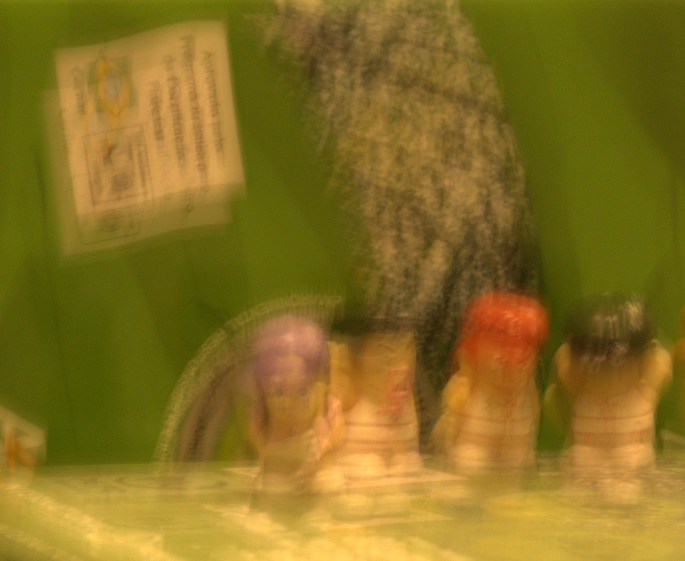输出图像：展开全文图像处理
• 对已知图像进行模糊处理,用逆滤波和维纳滤波恢复图像，有Matlab程序和自己试验后的结果及简要的分析讨论
• 在数学应用上，对于运动引起的图像模糊，最简单的方法是直接做...最小均方差（维纳滤波用来去除含有噪声的模糊图像，其目标是找到未污染图像的一个估计，使它们之间的均方差最小，可以去除噪声，同时清晰化模糊图像
在数学应用上，对于运动引起的图像模糊，最简单的方法是直接做逆滤波，但是逆滤波对加性噪声特别敏感，使得恢复的图像几乎不可用。最小均方差（维纳）滤波用来去除含有噪声的模糊图像，其目标是找到未污染图像的一个估计，使它们之间的均方差最小，可以去除噪声，同时清晰化模糊图像。

定义

给定一个系统
y(t)=h(t)∗x(t)+n(t)y(t)=h(t)*x(t)+n(t)
这里，∗$*$是卷积符号

x(t)$x(t)$是在时间t$t$刻输入的信号（未知）
h(t)$h(t)$是一个线性时间不变系统的脉冲响应（已知）
n(t)$n(t)$是加性噪声，与x(t)$x(t)$不相关（未知）
y(t)$y(t)$是我们观察到的信号
我们的目标是找出这样的卷积函数g(t)$g(t)$，这样我们可以如下得到估计的x(t)$x(t)$：
x^(t)=g(t)∗y(t)\hat x(t) = g(t)*y(t)
这里x^(t)$\hat x(t)$是x(t)$x(t)$的最小均方差估计。
基于这种误差度量， 滤波器可以在频率域如下描述
G(f)=H∗(f)S(f)|H(f)|2S(f)+N(f)=H∗(f)|H(f)|2+N(f)/S(f)\begin{split}
G(f) &= {{H^*(f)S(f)} \over {|H(f){|^2}S(f) + N(f)}}\\ &= {{H^*(f)} \over {|H(f){|^2} + N(f)/S(f)}}
\end{split}
这里：
G(f)$G(f)$和H(f)$H(f)$是g$g$和h$h$在频率域f$f$的傅里叶变换。
S(f)$S(f)$是输入信号x(t)$x(t)$的功率谱。
N(f)$N(f)$是噪声的n(t)$n(t)$的功率谱。
上标∗$*$代表复数共轭。
滤波过程可以在频率域完成：
X^(f)=G(f)∗Y(f)\hat X(f) = G(f)*Y(f)
这里 X^(f)$\hat X(f)$是 x^(t)$\hat x(t)$的傅里叶变换，通过逆傅里叶变化可以得到去卷积后的结果x^(t)$\hat x(t)$。

解释

上面的式子可以改写成更为清晰的形式
G(f)=1H(f)⎡⎣⎢|H(f)|2|H(f)|2+N(f)S(f)⎤⎦⎥=1H(f)⎡⎣|H(f)|2|H(f)|2+1SNR(f)⎤⎦\begin{split}
G(f) &= {1 \over {H(f)}}\left[ {{{|H(f){|^2}} \over {|H(f){|^2} + {{N(f)} \over {S(f)}}}}} \right] \\ &={1 \over {H(f)}}\left[ {{{|H(f){|^2}} \over {|H(f){|^2} + {1 \over {SNR(f)}}}}} \right]
\end{split}
这里H(f)$H(f)$是h$h$在频率域f$f$的傅里叶变换。SNR(f)=S(f)/N(f)$SNR(f)=S(f)/N(f)$是信号噪声比。当噪声为零时（即信噪比趋近于无穷），方括号内各项也就等于1，意味着此时刻维纳滤波也就简化成逆滤波过程。但是当噪声增加时，信噪比降低，方括号里面值也跟着降低。这说明，维纳滤波的带通频率依赖于信噪比。

推导

上面直接给出了维纳滤波的表达式，接下来介绍推导过程。
上面提到，维纳滤波是建立在最小均方差，可以如下表示：
e(f)=E|X(f)−X^(f)|2e(f) = E|X(f) - \hat X(f){|^2}
这里E$E$是期望
如果我们替换表达式中的X^(f)$\hat X(f)$，上面可以重新组合成
e(f)=E|X(f)−G(f)Y(f)|2=E|X(f)−G(f)[H(f)X(f)+V(f)]|2=E|[1−G(f)H(f)]X(f)−G(f)V(f)|2\begin{split}
e(f)  &= E|X(f) - G(f)Y(f){|^2} \\
&= E|X(f) - G(f)[H(f)X(f) + V(f)]{|^2}\\
&= E|[1 - G(f)H(f)]X(f) - G(f)V(f){|^2}
\end{split}
展开二次方，得到下式：
e(f)=[1−G(f)H(f)][1−G(f)H(f)]∗E|X(f)|2−[1−G(f)H(f)]G∗(f)E{X(f)V∗(f)}−G(f)[1−G(f)H(f)]∗E{V(f)X∗(f)}+G(f)G∗(f)E|V(f)|2\eqalign{
e(f)&= [1 - G(f)H(f)]{[1 - G(f)H(f)]^*}E{\rm{|X(f)}}{{\rm{|}}^2}  \cr
&- [1 - G(f)H(f)]{G^*}(f)E\{ X(f){V^*}(f)\}   \cr
&- G(f){[1 - G(f)H(f)]^*}E\{ V(f){X^*}(f)\}   \cr
&+ G(f){G^{\rm{*}}}(f)E|V(f){|^2}
\cr}
然而，我们假设噪声与信号独立无关，这样有
E{X(f)V∗(f)}=E{V(f)X∗(f)}=0E\{ X(f){V^*}(f)\} =E\{ V(f){X^*}(f)\}=0
并且我们如下定义功率谱
S(f)=E|X(f)|2N(f)=E|V(f)|2S(f) =E|X(f)|^2\\
N(f) =E|V(f)|^2
这样我们有
e(f)=[1−G(f)H(f)][1−G(f)H(f)]∗S(f)+G(f)G∗(f)N(f)\eqalign{
& e(f) = [1 - G(f)H(f)]{[1 - G(f)H(f)]^*}S(f) + G(f){G^*}(f)N(f)}
为了得到最小值，我们对G(f)$G(f)$求导，令方程等于零。
d(f)dG(f)=G∗(f)N(f)−H(f)[1−G(f)H(f)]∗S(f)=0{{{\rm d}(f)} \over {{\rm d}G(f)}} = {G^*}(f)N(f) - H(f){[1 - G(f)H(f)]^*}S(f) = 0
由此最终推出维纳滤波器。

测试

Matlab自带了示例程序，如下

%Read image
figure,subplot(2,3,1),imshow(I);
title('Original Image (courtesy of MIT)');

%Simulate a motion blur
LEN = 21;
THETA = 11;
PSF = fspecial('motion', LEN, THETA);
blurred = imfilter(I, PSF, 'conv', 'circular');
subplot(2,3,2),imshow(blurred);
title('Blurred Image');

%Restore the blurred image
wnr1 = deconvwnr(blurred, PSF, 0);
subplot(2,3,3),imshow(wnr1);
title('Restored Image');

%Simulate blur and noise
noise_mean = 0;
noise_var = 0.0001;
blurred_noisy = imnoise(blurred, 'gaussian', ...
noise_mean, noise_var);
subplot(2,3,4),imshow(blurred_noisy)
title('Simulate Blur and Noise')

%Restore the blurred and noisy image:First attempt
wnr2 = deconvwnr(blurred_noisy, PSF, 0);
subplot(2,3,5);imshow(wnr2);title('Restoration of Blurred, Noisy Image Using NSR = 0')

%Restore the Blurred and Noisy Image: Second Attempt
signal_var = var(I(:));
wnr3 = deconvwnr(blurred_noisy, PSF, noise_var / signal_var);
subplot(2,3,6),imshow(wnr3)
title('Restoration of Blurred, Noisy Image Using Estimated NSR');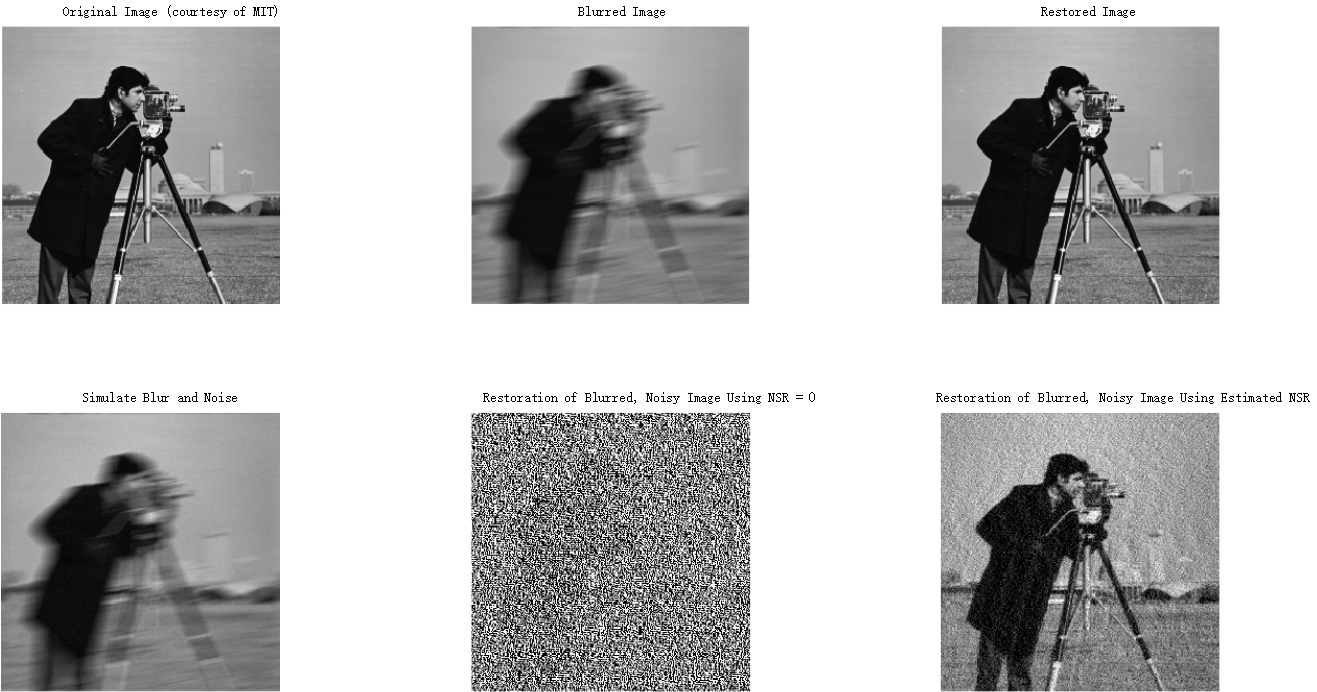维纳滤波需要估计图像的信噪比(SNR)或者噪信比(NSR)，信号的功率谱使用图像的方差近似估计，噪声分布是已知的。从第一排中可以看出，若无噪声，此时维纳滤波相当于逆滤波，恢复运动模糊效果是极好的。从第二排可以看出噪信比估计的准确性对图像影响比较大的，二排中间效果几乎不可用。

参考阅读

http://en.wikipedia.org/wiki/Wiener_deconvolution 英文维基百科
http://www.owlnet.rice.edu/~elec539/Projects99/BACH/proj2/wiener.html 莱斯大学的项目资料

转载保留声明

作者
日期
联系方式

风吹夏天
2015年5月29日
wincoder@qq.com


展开全文图像复原 维纳滤波
• 图像复原中的维纳滤波，逆滤波，运动模糊，效果很好，大家可以试试，移植简单
• 维纳滤波也称最小均方误差滤波，它能处理被退化函数退化和噪声污染的图像。该滤波方法建立在图像和噪声都是随机变量的基础之上，目标是找到未污染图像f(x,y)的一个估计，使它们之间的均方误差最小，即，其中E{.}是...
       维纳滤波也称最小均方误差滤波，它能处理被退化函数退化和噪声污染的图像。该滤波方法建立在图像和噪声都是随机变量的基础之上，目标是找到未污染图像f(x,y)的一个估计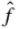，使它们之间的均方误差最小，即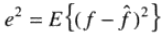，其中E{.}是参数期望值。在假设噪声和图像不相关，其中一个或另一个有零均值，且估计中的灰度级是退化图像中灰度级的线性函数的条件下，均方误差函数的最小值在频率域由如下表达式给出：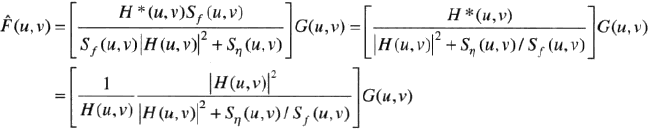其中，H(u,v)为退化函数，H*(u,v)为H(u,v)的复共轭，|H(u,v)|^2=H(u,v)H*(u,v)，Sη(u,v)=|N(u,v)|^2=噪声的功率谱，Sf(u,v)=|F(u,v)|^2=未退化图像的功率谱，H(u,v)是退化函数的傅里叶变换，G(u,v)是退化后图像的傅里叶变换。
从上面的公式可以发现，如没有噪声，即Sη(u,v)=0，此时维纳滤波变为直接逆滤波，如有噪声，那么Sη(u,v)如何估计将成问题，同时Sf(u,v)的估计也成问题。在实际应用中假设退化函数已知，如果噪声为高斯白噪声，则Sη(u,v)为常数，但Sf(u,v)通常难以估计。一种近似的解决办法是用一个系数K代替Sη(u,v)/Sf(u,v)，因此上面的公式变为如下式所示：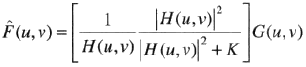在实际应用中，根据处理的效果选取合适的K值。
这里对256×256大小的灰度图像进行退化处理，然后添加均值为0，方差为0.005的高斯噪声，对得到的退化图像使用直接逆滤波和维纳滤波复原，并比较效果，MATLAB代码如下：
image_o=imread('C:\Program Files\MATLAB\R2013a\bin\work\图像复原\lena.bmp');
subplot(2,2,1);
imshow(image_o);
title('原图像');
%频率域退化图像，退化函数H(u,v)=exp(-0.0025*( (u-M/2).^2+(v-N/2).^2).^(5/6) )
%傅里叶变换
f=im2double(image_o);
F=fft2(f);
F=fftshift(F);
%执行退化
[M,N]=size(F);
[u,v]=meshgrid(1:M,1:N);%生成二维坐标系
H=exp(-0.0025* ( (u-M/2).^2+(v-N/2).^2).^(5/6) );
F=F.*H;
%添加高斯噪声
%傅里叶反变换
X=ifftshift(F);
x=ifft2(X);
x=uint8(abs(x)*256);
x1=imnoise(x,'gaussian',0,0.005);%添加高斯噪声
subplot(2,2,2);
imshow(x1);
%
title('退化图像');

%
Id=im2double(x1);
% 傅里叶变换
f_Id=fft2(Id);
f_Id=fftshift(f_Id);
fH_Id=f_Id;
D=abs(H);
D=D.^2;

[M1,N1]=size(fH_Id);
% 逆滤波
threshold=48;
K=0.08;
if threshold>M1/2
% 全滤波
fH_Id=fH_Id./(H+eps);
else
%对一定半径范围内进行滤波
for i=1:M1
for j=1:N1
if sqrt((i-M1/2).^2+(j-N1/2).^2)<threshold
% 维纳滤波公式
fH_Id(i,j)=fH_Id(i,j)./(H(i,j)) .* (D(i,j)./(D(i,j)+K));
end
end
end
end
% 执行傅立叶逆变换
fH_Id1=ifftshift(fH_Id);
f_new=ifft2(fH_Id1);
f_new=uint8(abs(f_new)*255);
subplot(2,2,3);
imshow(f_new);
title('维纳滤波复原图像');

fH_Id2=f_Id;
[M2,N2]=size(fH_Id2);
if threshold>M2/2
%全滤波
fH_Id2=fH_Id2./(H+eps);
else
%对一定半径范围内进行滤波
for i=1:M2
for j=1:N2
if sqrt((i-M2/2).^2+(j-N2/2).^2)<threshold
fH_Id2(i,j)=fH_Id2(i,j)./(H(i,j)+eps);
end
end
end
end

fH_Id3=ifftshift(fH_Id2);
f_new3=ifft2(fH_Id3);
f_new3=uint8(abs(f_new3)*255);
subplot(2,2,4);
imshow(f_new3);
title('直接逆滤波复原图像');处理效果如下图所示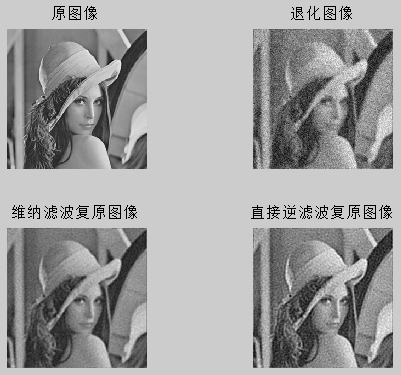从运行结果来看，维纳滤波性能优于直接逆滤波


展开全文matlab 图像处理 维纳滤波 图像复原
• 维纳滤波又称最小均方误差滤波，综合考虑了退化函数和噪声。均方误差由下式给出： e2=|f(x)−f^(x)|2 e^2=|f(x)-\hat f(x)|^2 假定噪声与图像是不相关的，复原图像的最佳估计可用下式表示： F^(u,v)=[HH(u,v)|H(u,...
逆滤波只能解决只有退化函数，没有加性噪声的问题。维纳滤波又称最小均方误差滤波，综合考虑了退化函数和噪声。均方误差由下式给出：
e2=|f(x)−f^(x)|2
e^2=|f(x)-\hat f(x)|^2

假定噪声与图像是不相关的，复原图像的最佳估计可用下式表示：
F^(u,v)=[HH(u,v)|H(u,v)|2+Sn(u,v)/Sf(u,v)]G(u,v)
\hat F(u,v)=\left[\frac{H^H(u,v)}{|H(u,v)|^2+S_n(u,v)/S_f(u,v)}\right]G(u,v)

因为 |H(u,v)|2=H(u,v)HH(u,v)$|H(u,v)|^2=H(u,v)H^H(u,v)$，因而可以写为：
F^(u,v)=[|H(u,v)|2|H(u,v)|2+Sn(u,v)/Sf(u,v)⋅1H(u,v)]G(u,v)
\hat F(u,v)=\left[\frac{|H(u,v)|^2}{|H(u,v)|^2+S_n(u,v)/S_f(u,v)}\cdot \frac1{H(u,v)}\right]G(u,v)

式中各项的定义分别如下：

（1）H(u,v)$H(u,v)$：退化函数
（2）HH(u,v)$H^H(u,v)$：共轭
（3）|H(u,v)|2=HH(u,v)H(u,v)$|H(u,v)|^2=H^H(u,v)H(u,v)$
（4）Sn(u,v)$S_n(u,v)$：噪声的功率谱
（5）Sf(u,v)$S_f(u,v)$：为退化函数的功率谱

观察公式可以发现，加入没有噪声，则 Sn(u,v)=0$S_n(u,v)=0$，此时维纳滤波退化为逆滤波。那么又该如何估计 Sn(u,v)/Sf(u,v)$S_n(u,v)/S_f(u,v)$ 呢。假设退化过程已知，则 H(u,v)$H(u,v)$ 可以确定，假如噪声为高斯白噪声，则 Sn(u,v)$S_n(u,v)$ 为常数，但 Sf(u,v)$S_f(u,v)$ 通常难以估计。一种近似的解决方法即是用一个系数 K$K$ 来代替 Sn(u,v)/Sf(u,v)$S_n(u,v)/S_f(u,v)$。此时公式变为：

F^(u,v)=[|H(u,v)|2|H(u,v)|2+K1H(u,v)]G(u,v)
\hat F(u,v)=\left[\frac{|H(u,v)|^2}{|H(u,v)|^2+K}\frac1{H(u,v)}\right ]G(u,v)

展开全文• 上一节讲了逆滤波，这一次讲讲维纳滤波，逆滤波在图像没有噪声的情况下是很好的，但在有噪声的情况下，噪声会被放大，所以维纳滤波就横空出世了，维纳滤波能很好的解决有噪声的图像修复。 维纳滤波是诺波特·维纳在...
上一节讲了逆滤波，这一次讲讲维纳滤波，逆滤波在图像没有噪声的情况下是很好的，但在有噪声的情况下，噪声会被放大，所以维纳滤波就横空出世了，维纳滤波能很好的解决有噪声的图像修复。
维纳滤波是诺波特·维纳在二十世纪四十年代提出的一种滤波器，即假定线性滤波器的输入为有用信号和噪声之和，两者均为广义平稳过程且知道它们的二阶统计特性，根据最小军方误差准则（滤波器的输出信号与需要信号之差的均方值最小），求得最佳线性滤波器的参数。
维纳滤波器是一种自适应最小均方差滤波器。维纳滤波的方法是一种统计方法，它用的最优准则是基于图像和噪声各自的相关矩阵，它能根据图像的局部方差调整滤波器的输出，局部方差最大，滤波器的平滑作用就越强。
公式如下：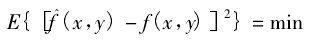其中戴帽子的f为我们维纳滤波后的图像，f为清晰的原始图像，g为待恢复的模糊图像（g上式没有，写出来为了说明的完整性）。
这里戴帽子的f我们是不知道的，所以我们要根据这个优化式子求出$\hat{f}$.
具体的推导过程这里不写，因为我也只看了个大概。
求解的结果为：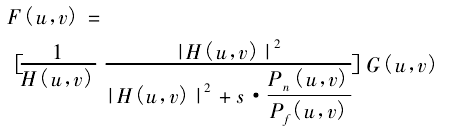其中，G(u,v)是退化图形的傅里叶变换；
H(u,v)是退化函数；
$p_n(u,v)=|N(u,v)|^2$是噪声的功率谱；
$P_f(u,v)=|F(u,v)|^2$是原始图像的功率谱；
$s=\frac{1}{λ}$,λ为一常数，是拉格朗日乘数。
之前有说过维纳滤波需要知道原始图像和噪声的二阶统计特性，即要知道关于图像和噪声的先验知识，比如$p_f(u,v),p_n(u,v)$，但是这恰恰也是我们不知道的，这也是维纳滤波器的局限所在，我们一般将上述两个公式的比值看做是常数带入进行计算，如下所示：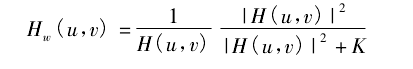这是一种无可奈何的粗糙的近似，但是当噪声为白噪声即其功率谱为常数的时候，这种近似效果很不错。
为了强化维纳滤波器的效果“基于维纳滤波的图像复原”这篇论文提出了两次维纳滤波的方法，第一次维纳滤波求出一个近似图像，然后由近似图像的和模糊图像估计噪声和原始图像的先验知识，最后根据该先验知识进行再次的维纳滤波。
“Lucy - Ｒichardson 与维纳滤波算法比较分析”，这篇文章指出在先加模糊函数在加噪声的情况下，维纳滤波的表现比LR滤波器的表现更为良好。
在“维纳滤波图像复原技术的研究与改进”这篇文章中，作者指出，维纳算法可实现最小均方差复原，当图像的频率特性和噪声已知时，维纳滤波的效果较好；在峰值信噪较低时效果不好。这时由于三个问题限制了它的有效性。首先维纳滤波采用均方误差（MSE）准则，该准则对所有的误差（不管其在图像中的位置）都赋予同样的权，而人眼对暗处和高梯度区域的误差比其他区域的误差有较大的容忍性，所以，维纳滤波以一种并非适合人眼的方式对图像进行了平滑（第一个公式即求均方误差最小）；其次，维纳滤波假设退化模型为线型空间不变系统，它不能处理具有空间可变点扩散函数的情形（比如物体运动不是匀速或者匀加速）；最后，由于基于平稳随机场的模型，维纳滤波 不能处理有着非平稳信号和噪声的一般情形，大部分图像都是高度非平稳的，有着陡峭边缘分开的大块平坦区域（对非平稳过程而言，均值和方差是无法通过统计得到的，因为它们会随时间而变化）。
参考文献：
Lucy - Ｒichardson 与维纳滤波算法比较分析
维纳滤波图像复原技术的研究与改进
最后推荐一篇非常不错的维纳滤波的博文：https://blog.csdn.net/baimafujinji/article/details/73882265


展开全文• 目的：对获取图像在频域用高斯函数进行退化并叠加白噪声，对退化图像进行逆滤波和维纳滤波恢复，比较原始图像和恢复图像，对利用逆滤波和维纳滤波恢复方法恢复图像进行比较。 一、基本原理  图像复原是一种客观的...
• 图像降噪算法——维纳滤波图像降噪算法——维纳滤波 图像降噪算法——维纳滤波计算机视觉 图像降噪 数字图像处理
• 维纳滤波法是一种统计方法，是建立在图像和噪声都是随机过程，图像和噪声不相关的基础上，由此得到的结果在图像统计平均意义下是最优的。本文将深入研究著名的维纳滤波，特别是其背后的数学原理。这也涉及到了限制性...维纳滤波 图像复原 图像处理 matlab
• 摘 要：本实验主要使用维纳滤波法（又名为最小均方误差滤波）实现图像复原与重建。首先我们通过对一幅图像加入运动污损滤波和高斯噪声，然后从噪声中提取出原始图像信号。在各种估计方法中，维纳滤波是一种最基本的...c matlab filter 维纳滤波
• 内容简介运动模糊核方向和角度估计最优运动模糊核长度和角度确定运动模糊核生成维纳滤波 运动模糊核方向和角度估计 这里介绍一种通用的做法即倒谱如下： 这里需要注意的是公式中的I 是傅立叶变换后的其他的就是...python 算法 图像识别 opencv
• 图像复原代码。运动模糊图像复原、非约束复原、逆滤波复原、维纳滤波复原等
• 这是用matlab实现图像复原的程序，包括逆滤波、维纳滤波、约束最小二乘方滤波。
• 对一副数字图像模拟出运动模糊效果并采用维纳滤波；模拟出大气湍流效果并采用逆滤波。MATLAB r2013a。
• 在MATLAB中采用均方误差和测度两种评价参数对维纳滤波和L-R算法复原的加入噪声的运动模型和高斯模型的图像复原效果进行了比较
• 参考资料： 陷波滤波器—matlab实现 http://blog.sina.com.cn/s/blog_ebd29d830102wdzw.html 图像复原之约束最小二乘方滤波 ... 图像模糊（约束最小二乘方滤波） https://blog.csdn.net/blu...维纳滤波 约束最小二乘滤波 matlab 图像处理
• 维纳滤波（wiener filtering) 一种基于最小均方误差准则、对平稳过程的最优估计器。这种滤波器的输出与期望输出之间的均方误差为最小，因此，它是一个最佳滤波系统。可用于提取被平稳噪声所污染的信号。 　从连续的...维纳滤波 matlab
• 逆滤波与维纳滤波一、算法描述1.运动模糊主要使用课本公式: H(u, v) = sin(pi * (a * u + b * v)) * exp(-1i * pi * (a * u + b * v)) * T / (pi * (a * u + b * v))。但另外需注意图像矩阵f在傅里叶变换前和傅里叶...数字图像处理
• 利用逆滤波和维纳滤波，对Lena加噪运动模糊降质图像进行复原，比较不同参数选择对复原结果的影响。二、实验内容1)     输入Lena图像，对图像进行运动降质；降质模型：2) ...
...